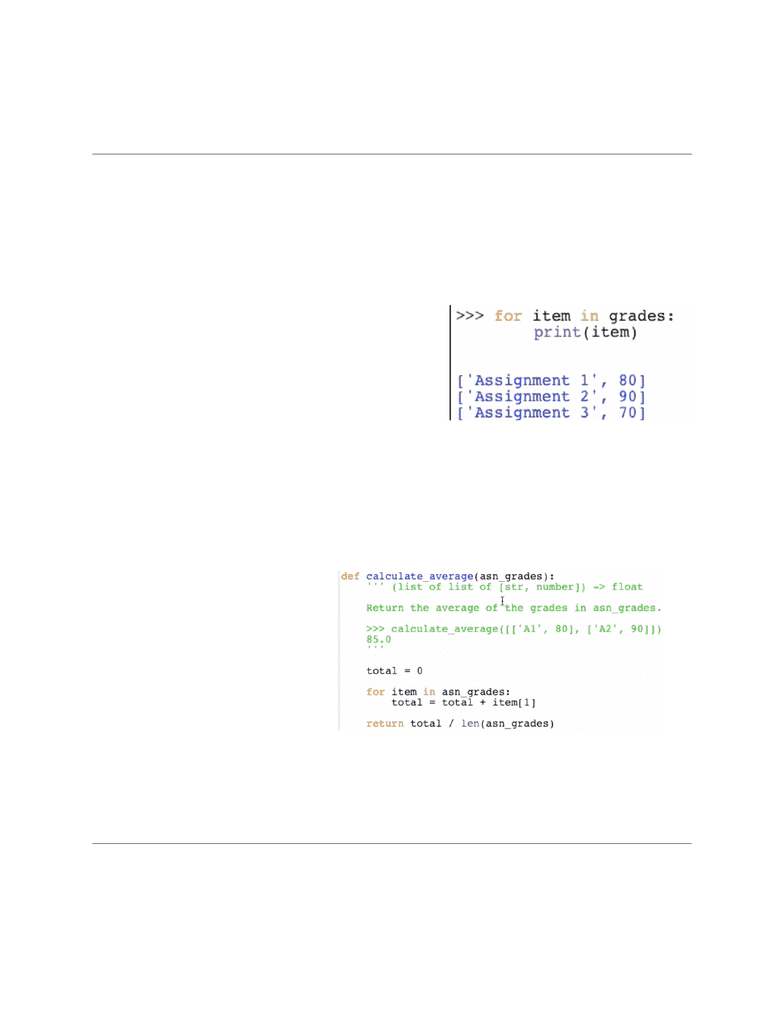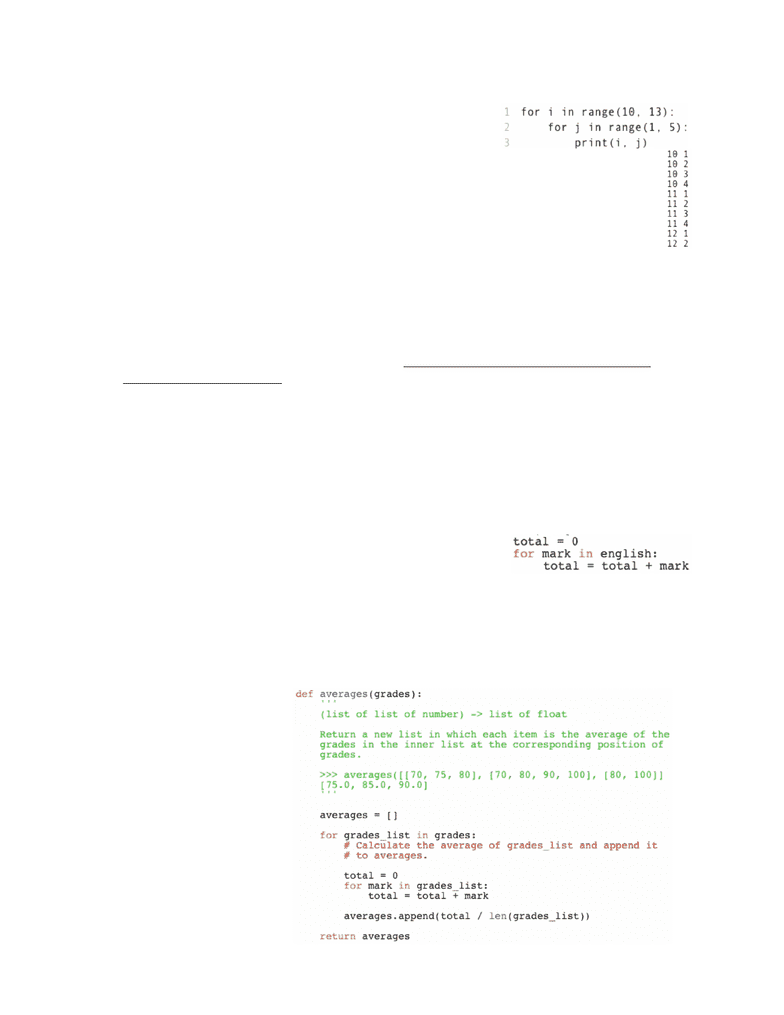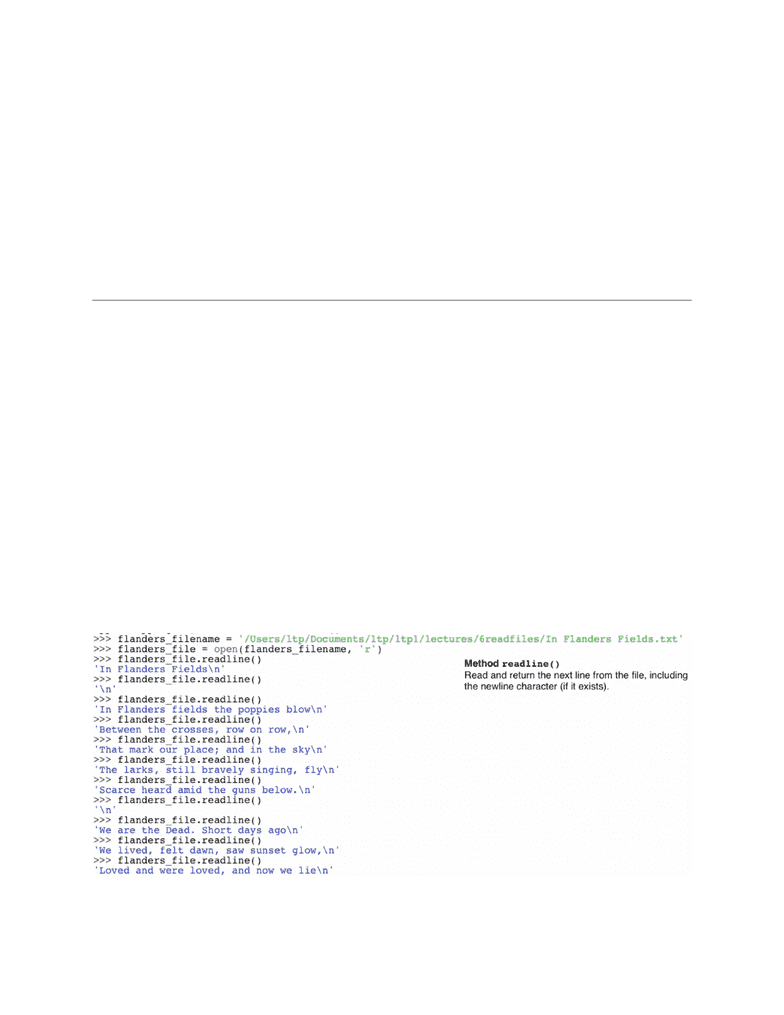Class Notes (1,100,000)
CA (620,000)
UTSC (30,000)
All (10)
Lecture 6

# CSCA08 Lecture 6: Week 6 + 7 Notes

Department
Computer Science
Course Code
CSCA08H3
Professor
All
Lecture
6

This preview shows pages 1-3. to view the full 12 pages of the document.Alexander Magony
CSC108H1F 2014
Week 6
Nested Lists
-Nested lists: lists that contain a list as an item within itself.
eg. grades = [[‘Assignment 1’, 80], [‘Assignment 2’, 90], [‘Assignment 3’, 70].
-This list is a three item list. Each item is a list with two elements.
len(grades) returns 3.
grades returns [‘Assignment 1, 80], the list at index 0 within the grades nested list.
len(grades) returns 2 since the item at that index is a list with two items. The length
of each of these inner lists is 2.
-Using a for loop to print each item in this
nested list would again demonstrate that each
item within the grades list is another list.
-These inner lists can be accessed by index
as well.
eg. grades returns the str ‘Assignment
1’. This is because the item at index 0 of
grades is a list and the item at index 0 of this
ﬁrst item of grades is a string. So basically, you’re accessing the item at index 0 of the
list at index 0 of grades.
eg. grades would return 90.
-Problem-solving: writing a function that returns the average of all of the marks of a nested
list which contains lists which contains two elements (a string with the assignment name and
a number of the mark). Basically what we’ve been looking at in our above example.
Since the marks are
represented as the second item
of each list within the nested
list, we want to average the
values that belong at index 1 of
each list within the nested list.
So for each item in the nested
loop (ie. the inner two-element
list), we want to add the value
found at index 1 of the item (ie.
the number representing the
mark) to an accumulator
variable called total. As each inner list is considered, each mark will be added to the total.
Then we divide the total by the number of items in the nested list by dividing by the length
of the nested list and return this value.
Nested Loops
-For loops can also be created inside other for loops.
1

Only pages 1-3 are available for preview. Some parts have been intentionally blurred.Alexander Magony
CSC108H1F 2014
In the example to the right, for every i in range 10 to but
not including 13, a inner for loop will be executed looking
at every j in range 1 to but not including 5.
So, ﬁrst i will equal 10. Now we go inside that for loop and
look at the next for loop. j ﬁrst equals 1. So we print 10 1. Since the inner for loop is
still within its range, we now consider j equaling 2. So we print 10 2.
We will continue until after we print 10 4. This is the last value within the range of the
inner for loop. So now we return to the outer for loop and i now equals 11. Now we
return to the inner for loop and repeat the process but with i equaling 11.
After we ﬁnish the range of the j for loop, we return back to the i loop and i equals
12. Since this is the last value to be considered in the outer loop, we will exit this for loop
after its contents (the inner for loop) are completed.
A picture of the printed output is shown below the picture of the function, except 12 3 and
12 4 would also be printed after what is shown.
This is all shown within a visualizer so, if you’d like to see how the variables and values
are saved and created, watch the ﬁrst video: https://teach.cdf.toronto.edu/StG108/
content/challenges/83/1
-Problem-solving: return a list of the averages in each of the inner lists of grades. In this
example, grades refers to [[70,75,80], [70,80,90,100], [80, 100]].
First we need to consider the subproblem of how to calculate the average of a list of
values. So let’s say the english variables refers to grades, the ﬁrst list of variables in
grades ([70,75,80]). To calculate the average, we need to add up all of the individual items
of this list to an accumulator variable total and then divide by len(grades), the length of
this list.
-To actually add each item in this list, we need to write the
for loop shown to the right. For each mark in english (in
this case referring to a list within the list grades at index
0. In the ﬁnal function we will probably use a different
variable), we add the mark to the total. After this is done
for each of the three marks, total will refer to 225. Dividing by the length of the list (3) will
return the average 75.0.
This needs to be done for each list in the nested list grades and these averages will need
to be assembled into a new list that is returned.
Since the average of the
ﬁrst list is 75.0, the
second list is 85.0, and
the third list is 90.0, we
expect a returned list of
averages [75.0, 85.0,
90.0]. They are all ﬂoats
since we are dividing
normally. In other words,
a list of list of number
returns a list of ﬂoat (the
type contract).
averages will be the
empty list that will
accumulate the average
2

Only pages 1-3 are available for preview. Some parts have been intentionally blurred.Alexander Magony
CSC108H1F 2014
of each list in grades.
We ﬁrst need to create a for loop that considers each grades_list in grades. For each
grade_list, we need to calculate its average and append it to the averages list.
We can use the for loop we created above for this subproblem (of ﬁnding the average for
each list). After the average is calculated with this for loop we’ve created above, we need to
append that average to the averages list. Since this is all done in the for loop considering
each grades_list in grades, the average is calculated for each grades_list (using the inner
for loop) and appended to the growing averages list. The averages list is then returned.
Week 7
Reading Files
-So far our computer programs were used to store information. Most information is stored in
ﬁles on our computer. We have four ways to read a text ﬁle.
-Before we read a ﬁle, we must open it with Python.
-open(ﬁlename, mode). The modes include:
-‘r to open for reading
-‘w’ to open for writing (erasing what is already in the ﬁle).
-‘a’ to open for appending (adding to what is already in the ﬁle)
Regarding the ﬁlename:
-if the ﬁlename is in the same folder as the Python program, we just write the ﬁlename.
-If it is in a different folder, we must give the full path to it (eg. ‘/Users/ltp/Documents/ltp/
ltpl/lectures/6readﬁles/In Flanders Fields.txt’). We usually save the ﬁle name to a
variable so we don't have to retype it.
-1. readline approach: Using the In Flanders Fields.txt example, we can use method
readline() to read the ﬁrst line of the text ﬁle after we open it. This includes the \n at the end
of the line. If we keep calling this exact method, we will read through each line of the poem
one at a time. Look at the below picture of the video to see this play out.
3
###### You're Reading a Preview

Unlock to view full version